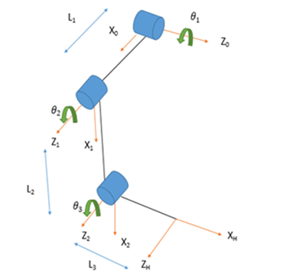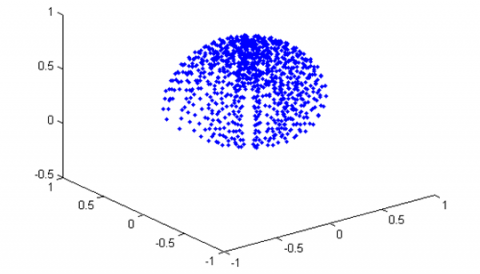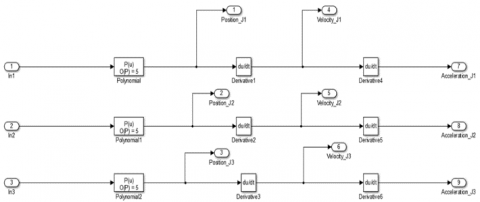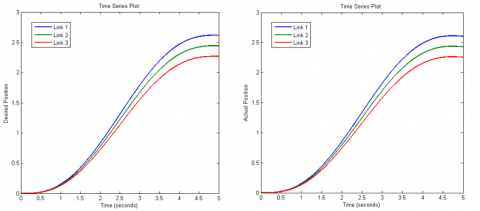# Kinematic, Dynamic Analysis and Control of 3 DOF Upper-limb Robotic Exoskeleton

Kinematic, Dynamic Analysis and Control of 3 DOF Upper-limb Robotic Exoskeleton
University of Petroleum and Energy Studies, Dehradun 248007, India

Manipal Academy of Higher Education, Dubai 345050, UAE

Corresponding Author Email:
akash.gupta@ddn.upes.ac.in
Page:
297-304
|
DOI:
https://doi.org/10.18280/jesa.520311
15 March 2019
|
Accepted:
28 May 2019
|
Published:
31 August 2019
| Citation

OPEN ACCESS

Abstract:

The purpose of this paper is to analyse complete kinematics and dynamics, along with the joint position analysis of a 3 DOF upper-limb robotic exoskeleton. This paper also investigates the feasibility of computed torque control for an exoskeleton device. After studying the biomechanics of human upper-limb, a 3 DOF exoskeleton has been designed. The development of upper limb and lower extremity robotic exoskeletons has emerged as a way to improve the quality of life as well as act as a primary rehabilitation device for the individuals suffering from stroke or spinal cord injury. The designed exoskeleton presents three of the most basic movements of the human arm that facilitate activities of daily living (ADL). The design parameters are taken similar to the parameters of the upper-limb of a normal human being. Computed torque control (CTC) is applied to the system in order to actuate the system to the desired joint positions. The exoskeleton exhibits shoulder abduction/adduction, extension/flexion and elbow extension/flexion motions. For better understanding of exoskeleton motion capabilities, the exoskeleton workspace is visualized and the workspace is obtained using MATLAB. The results clearly show that the CTC control successfully reduces the error in the exoskeleton joint positions.

Keywords:

robotic rehabilitation, computed torque control, upper-limb, joint trajectory, robot dynamics

1. Introduction

Robotic exoskeletons are mostly designed for the purpose of power augmentation, assistance training and rehabilitation purposes. These wearable exoskeletons are quite different from end effector based training robots because end-effector based robots interact with patients through one point only  while the wearable exoskeletons have multiple contact points closely resembling the structure of human limb. Even today, most of the limb rehabilitation is being done manually with the help of physiotherapy sessions. These sessions lack repeatability and are short. With the help of automated robotic rehabilitation therapy, these sessions can be easily extended with better repeatability and are far less tedious. There are several studies that provide sufficient data on effectiveness of robotic based rehabilitation therapy on rehabilitation progress of stroke patients . Through last decade, with the advancement of robotic technology, the robotic assistive, rehabilitative therapy has emerged with much greater potential for the future. There has been continuous research in this field in order to achieve better human-robot interaction. The proposed design is a 3DOF active exoskeleton.

Due to the complexity of shoulder joint also known as Glenohuemral (GH) joint, the proposed model has simple 2DOF motion at the shoulder joint namely abduction/adduction and extension/flexion. The upper-limb plays a very special part in our activities of daily living (ADL) and has altogether 37 bones . The wide range of motion in the human upper-limb is due to the fact that upper-limb has 30DOF. The proposed exoskeleton closely resembles the structure of human upper-limb with all revolute joints. Considering the accuracy, repeatability, cost and time, human assisted rehabilitation process lags behind robotic assisted rehabilitation . The purpose of proposed robotic exoskeleton is to develop a comparatively simple robotic exoskeleton that can perform three of the most basic movements in the human upper-limb as explained earlier. This paper also validates the feasibility of CTC control for a 3DOF active exoskeleton device.

The following content is divided into 4 parts. Section 2 consists of kinematic analysis that further includes workspace analysis. Section 3 provides the dynamic analysis of the exoskeleton robot along with the workspace calculation. Section 4 consists of the implementation of computed torque control (CTC) and section 5 consists of the simulation results. Section 6 contains concluding remarks.

2. Kinematic Analysis

The kinematic analysis consists of forward and inverse kinematics of the robotic system. The forward kinematics provides the coordinates of the point in space that the end effector of the system achieves while knowing the joint angles. With inverse kinematics, the desired point in the workspace is known and the joint angles are calculated.

2.1 Forward kinematic analysis of 3-DOF upper-limb exoskeleton

The forward kinematics is obtained after calculating the dh-parameters of the proposed robotic exoskeleton device. The proposed design has similarity with the human upper-limb. The kinematic model of the design is shown in Figure 1. The D-H parameters of the model are shown in Table 1.

Table 1. DH parameters of 3-DOF upper-limb exoskeleton

 # Ɵ d a α 0-1 Θ1 L1 0 90֯ 1-2 Θ2 0 L2 0֯ 2-H Θ3 0 L3 0֯

## 1.pngFigure 1. Kinematic configuration of exoskeleton device

The Transformation matrix of each link is as follows:

$A_{1}=\left[\begin{array}{cccc}{\cos \theta_{1}} & {-\sin \theta_{1}} & {0} & {0} \\ {\sin \theta_{1}} & {\cos \theta_{1}} & {0} & {0} \\ {0} & {0} & {1} & {0} \\ {0} & {0} & {0} & {1}\end{array}\right] \times$$\left[\begin{array}{cccc}{1} & {0} & {0} & {0} \\ {0} & {1} & {0} & {0} \\ {0} & {0} & {1} & {l_{1}} \\ {0} & {0} & {0} & {1}\end{array}\right] \times\left[\begin{array}{cccc}{1} & {0} & {0} & {0} \\ {0} & {1} & {0} & {0} \\ {0} & {0} & {1} & {0} \\ {0} & {0} & {0} & {1}\end{array}\right] \times\left[\begin{array}{cccc}{1} & {0} & {0} & {0} \\ {0} & {\cos 90} & {-\sin 90} & {0} \\ {0} & {\sin 90} & {\cos 90} & {0} \\ {0} & {0} & {0} & {1}\end{array}\right]$$=\left[\begin{array}{cccc}{-\sin \theta_{1}} & {0} & {\cos \theta_{1}} & {-l_{1} \sin \theta_{1}} \\ {\cos \theta_{1}} & {0} & {\sin \theta_{1}} & {l_{1} \cos \theta_{1}} \\ {0} & {1} & {0} & {0} \\ {0} & {0} & {0} & {1}\end{array}\right]$

Similarly,

$A_{2}=\left[\begin{array}{cccc}{\cos \theta_{2}} & {-\sin \theta_{2}} & {0} & {l_{2} \cos \theta_{2}} \\ {\sin \theta_{2}} & {\cos \theta_{2}} & {0} & {l_{2} \sin \theta_{2}} \\ {0} & {0} & {1} & {0} \\ {0} & {0} & {0} & {1}\end{array}\right]$

$A_{3}=\left[\begin{array}{cccc}{-\sin \theta_{3}} & {-\cos \theta_{3}} & {0} & {-l_{3} \sin \theta_{3}} \\ {\cos \theta_{3}} & {-\sin \theta_{3}} & {0} & {l_{3} \cos \theta_{3}} \\ {0} & {0} & {1} & {0} \\ {0} & {0} & {0} & {1}\end{array}\right]$

$A_{hand}=A_{1} \times A_{2} \times A_{3}$

where, Ahandshows the position and orientation of the end effector of robotic exoskeleton.

2.2 Exoskeleton workspace

In order to calculate the workspace from the forward kinematics of the robotic system, a MATLAB code for all the reachable points of the end effector is developed. Figure 2 shows all the reachable points by the end effector of the robotic exoskeleton.

## 2.pngFigure 2. 3-DOF exoskeleton workspace

Robotic workspace typically shows the orientation and position of the end effector on different locations within its reach , which entirely depends upon the forward kinematics and joint angle (θ) range of each joint. In order to generate the 3D plot, joint positions of the end effector (Px, Py, Pz) are required.

3. Dynamic Analysis

3.1 Dynamic modelling of 3-DOF exoskeleton

The exoskeleton joint torques are calculated using Lagrangian, L=K-P. Where, P is the potential energy and K is kinetic energy of the system.

$L=\frac{1}{2} \sum_{i=1}^{n} \sum_{j=1}^{i} \sum_{k=1}^{i} \operatorname{Tr}\left[\left(^{0} T_{j-1} Q_{j}^{j-1} T_{i}\right) I_{i}\left(^{0} T_{k-1} Q_{k}^{k-1} T_{i}\right)^{T}\right] \stackrel{\cdot}{q}_{j}\stackrel{\cdot} q_{k}+\sum_{i=1}^{n} m_{i} g^{0} T_{i}^{i} \overline{r}_{i}$  (1)

According to the Euler-Lagrange dynamic formulation, τ is the generally termed as torque at joint i, that drives link i of the manipulator. It is given by

$\tau_{i}=\frac{d}{d t}\left(\frac{\delta L}{\delta \dot{q}_{i}}\right)-\frac{\delta L}{\delta q_{i}}$     (2)

Torque τi is applied to link i after carrying out the differentiation.

$\tau_{i}=\sum_{j=1}^{n} M_{i j}(q) \stackrel{\cdot}{q_{j}}+\sum_{j=1}^{n} \sum_{k=1}^{n} h_{i j k} \stackrel{\cdot}q_{j} \stackrel{\cdot}q_{k}+G_{i}$  for i = 1,2,…,n  (3)

where,

$M_{i j}=\sum_{p=\max (i, j)}^{n} \operatorname{Tr}\left[d_{p j} I_{p} d_{p i}^{T}\right]$  (4)

$h_{i j k}=\sum_{p=\max (i, j, k)}^{n} \operatorname{Tr}\left[\frac{\partial\left(d_{p k}\right)}{\partial q_{p}} I_{p} d_{p i}^{T}\right]$   (5)

$G_{i}=-\sum_{p=i}^{n} m_{p} g d_{p i}^{p}\overline r_{p}$  (6)

also

$d_{i j}=\left\{\begin{array}{ll}{^{0} T_{j-1} Q_{j}^{j-1} T_{i}} & {\text { for } j \leq i} \\ {0} & {\text { for } j>i}\end{array}\right.$  (7)

$\frac{\partial d_{i j}}{\partial p_{k}}=\left\{\begin{array}{ll}{^{0} T_{j-1} Q_{j}^{j-1} T_{k-1} Q_{k}^{k-1} T_{i}} & {\text { for } \mathrm{i} \geq \mathrm{k} \geq \mathrm{j}} \\ {^{0} T_{k-1} Q_{k}^{k-1} T_{j-1} Q_{j}^{j-1} T_{i}} & {\text { for } \mathrm{i} \geq j \geq \mathrm{k}} \\ {0} & {\text { for } \mathrm{i}<j \text { or } \mathrm{i}<\mathrm{k}}\end{array}\right.$  (8)

The 3-DOF upper-limb exoskeleton consisting of all revolute joints and link lengths l1=180 mm, l2=260 mm, l3=385 mm as per the measurement of an individual with height 189 cm. Link l2 and l3 have variable lengths in the form of a sliding mechanism that can shorten or lengthen the links associated with upper arm and forearm. The links are made up of aluminum alloy 6061. The joint torque is calculated after applying the payload in the form of arm weight on each link. For a healthy individual with weight 70 kg, the entire mass of the upper arm is 3.47kg that include the weight of the Forearm–1.12 kg; Upper arm – 1.89 kg and Hand – 0.46 kg. kg .

3.2 Trajectory planning

Trajectory is the sequence of motions of the joint with respect to time . The trajectory of the robot depends both on the dynamics and kinematics of the robot. Link 1 is fixed on the rear side of shoulder joint to facilitate abduction/adduction motion. The range of motion of human upper-limb for the shoulder abduction/adduction, extension/flexion and elbow extension/flexion is given in Table 2.

Table 2. Movement range of required upper-limb motions [8-9]

 Upper-limb movement Movement range Exoskeleton range Shoulder abduction/adduction 180°/0° 150° Shoulder flexion/extension 150°-180°/-40° to -50° 140° Elbow flexion/extension 135°-140°/0° 130°
For safety of the patient/wearer only positive motions (shoulder abduction, shoulder flexion, elbow flexion/extension) is considered. The range of movement varies from one individual to another. The exoskeleton movement range of different links is set below the maximum range of human upper-limb as per the biomechanics of human arm. To achieve a smooth and continuous motion, fifth order trajectory has been designed.

$\theta_{1}=12 t^{3}-3.6 t^{4}+0.2880 t^{5}$

$\theta_{2}=11.2 t^{3}-3.36 t^{4}+0.2688 t^{5}$

$\theta_{3}=10.4 t^{3}-3.12 t^{4}+0.2496 t^{5}$     (9)

4. Control System Design

It is well known that robotic systems are highly nonlinear , complicated and dynamically coupled . Without the implementation of a suitable control technique, the non-linearity of robotic systems results in uncertainty. The Computed Torque Control (CTC) has been employed into the system. The globally asymptotically stability of CTC makes it very effective motion control system . The controller in CTC modifies the system to effectively decouple and linearize by employing a nonlinear feedback of joint velocities and its actual positions . The schematic representation of the control system is shown in Figure 3.

## 3.pngFigure 3. Computed torque control law for nonlinear controller

The joint torques τ based on the rigid body dynamics.

$\tau=M(q) \stackrel{\cdots}{q}+H(q, \dot{q})+G(q)$   (10)

The contributions of overall non-rigid body effects and frictions, $F(q, \dot{q})$ has been neglected in the system. The errors in the controller are defined as

\begin{aligned} E(t) &=q_{d}(t)-q(t) \\ \stackrel{\cdot}E(t) &=\stackrel{\cdot}q_{d}(t)-\stackrel{\cdot}q(t) \end{aligned}  (11)

where, q and qd are actual and desired positions of the robot. Kp and Kd are position and velocity gains.

Though the links are assumed to be rigid bodies, the flexibility of the links very much constrains the selection of control gains . All mechanical elements produce resonance at frequencies other than natural frequency due to unmodelled structural flexibility. The controller must be designed in such way to avoid excitation of these unmodelled resonances.

$\omega_{r e s}=\omega_{0} \sqrt{\frac{I_{0}}{I_{\max }}}$    (12)

where, ωres is the resonance frequency, ω0 is the actual structural frequency at I0which is effective inertia. The controller must prevent the excitation of the design above natural frequency ωn to ensure the structural stability. The natural frequency is given by

$\omega_{n} \leq 0.5 \omega_{r s}$     (13)

Also

$\omega_{n}=\sqrt{K_{p}}$   (14)

From Eq. (12), we have

$\omega_{n} \leq 0.5 \omega_{0} \sqrt{\frac{I_{0}}{I_{\max }}}$   (15)

Therefore,

$K_{p} \leq\left(0.5 \omega_{0}\right)^{2} \frac{I_{0}}{I_{\max }}$   (16)

The Computed Torque Control (CTC) employs uses nonlinear feedback in order to linearize the error dynamics. This in turn provides better trajectory tracking performance. The Simulink model of joint trajectory is shown in Figure 4.

## 4.pngFigure 4. Joint trajectory Simulink model

5. Simulation Results

Simulations were carried on Matlab and Simulink (version 2014a). Figure 5 shows the desired and the actual joint positions of the 3-DOF exoskeleton after the application of control system. Figure 6 shows the comparison of required and actual joint trajectories before the application of CTC control scheme. The simulation clearly shows the final joint positions missing the required values by a great margin. The comparison of both as shown in Figure 5 and Figure 7 clearly validates the performance of CTC control system for the proposed design.

Figure 8 shows the designed controller that employs the basic principles of the computed torque control (CTC). Figure 9 shows the error comparison.

## 5.png(a) Desired joint positions                      (b) Actual joint positions

Figure 5. Desired joint positions and actual joint positions

## 6.pngFigure 6. Comparison of actual and required joint trajectories of 3-DOF exoskeleton before application of CTC scheme

## 7.pngFigure 7. Comparison of actual and desired positions after application of CTC

## 8.pngFigure 8. CTC designed in simulink for 3-DOF robotic exoskeleton

## 9.pngFigure 9. Joint position error without application of CTC and with CTC control

6. Conclusion

The purpose of this research is to develop a simple 3 DOF robotic exoskeleton and validate the required joint positions using Computed Torque Control (CTC). Kinematic and dynamic assessments are performed on the proposed system.

The exoskeleton performs the most basic movements of human upper-limb that are required in the activities of daily living (ADL). For the purpose of viability and real life situation, the external loads applied on the exoskeleton are the actual parameters of the normal human being. The joint parameters of the exoskeleton are matched with the joint parameters of the human beings. Verified by simulation results, the model with CTC shows accurate tracking of the joints. Also, the results show that CTC control optimally reduces the errors that are present in the output of the system when the simulation is done without the application of CTC control. Further research is required in the field of exoskeleton by improving human-robot interaction (HRI), accuracy of robotic devices. From the beginning of design phase, real life applications must be taken into consideration for the rehabilitation/assistive robots. These real life applications must include activities of daily living (ADL). The development of the working prototype of the proposed system is in the initial stages and employs surface electromyography (sEMG) sensors for the purpose of grasping human intentions and motor actuation. The Exoskeleton will employ CTC control in order to achieve the required joint positions.

Acknowledgement

This research is supported by University of Petroleum and Energy Studies, Research and Development under SEED grant UPES/R&D/07022019/11.

Nomenclature
 Px, Py,Pz Robot position coordinates iri Position Vector 0Ti Transformation Matrix L K Lagrangian Kinetic Energy P Potential Energy m Mass, Kg g I q $\dot{\mathrm{q}}$ $\ddot{\mathrm{q}}$ Mij hijk Gi τi t Kp Kd Greek Symbols α Ɵ ω Abbreviations D-H CTC DOF GH ADL Tr sEMG Acceleration due to gravity, m-s-1 Inertia Tensor Ɵ (Joint Displacement) Joint Velocity Joint Acceleration Mass matrix Coriolis Matrix Gravity Matrix Torque at link i, N-m Time Position gain Velocity gain   Angle about the common normal Theta Frequency   Denavit-Hartenberg Computed Torque Control Degree of Freedom Glenohumeral Activities of Daily Living Trace Surface Electromyography

APPENDIX

$Q_{1}=\left[\begin{array}{cccc}{0} & {-1} & {0} & {0} \\ {1} & {0} & {0} & {0} \\ {0} & {0} & {0} & {0} \\ {0} & {0} & {0} & {0}\end{array}\right]$,

$Q_{2}=\left[\begin{array}{cccc}{0} & {-1} & {0} & {0} \\ {1} & {0} & {0} & {0} \\ {0} & {0} & {0} & {0} \\ {0} & {0} & {0} & {0}\end{array}\right]$,

$Q_{3}=\left[\begin{array}{cccc}{0} & {-1} & {0} & {0} \\ {1} & {0} & {0} & {0} \\ {0} & {0} & {0} & {0} \\ {0} & {0} & {0} & {0}\end{array}\right]$

$I_i=\left[\begin{array}{cccc}{\frac{1}{2}\left(-I_{x x}+I_{y y}+I_{zz}\right)} & {I_{xy}} & {I_{x}} & {m_i\overline{x_i}} \\ {I_{x y}} & {\frac{1}{2}\left(I_{x x}-I_{yy}+I_{zz}\right)} & {I_{y z}} & {m_i\overline{y_i}} \\ {I_{x}} & {I_{y z}} & {\frac{1}{2}\left(I_{x x}+I_{y y}-I_{zz}\right)} & {m_i\overline{z_i}} \\ {m_i \overline{x_{i}}} & {m_i\overline{y_i}} & {m_i\overline{z_i}} & {m_i}\end{array}\right]$

$d_{11}=A_{0} Q_{1} A_{1}, d_{21}=A_{0} Q_{1} A_{1} A_{2}, d_{31}=A_{0} Q_{1} A_{1} A_{2} A_{3}$,

$d_{22}=A Q_{2} A_{2}, d_{32}=A_{1} Q_{2} A_{2} A_{3}, d_{33}=A_{1} A_{2} Q_{3} A_{3}$

$m_{11}=\operatorname{trace}\left(d_{11} I_{1} d_{11}\right)+\operatorname{trace}\left(d_{21} I_{2} d_{21}\right)+\operatorname{trace}\left(d_{31} I_{3} d_{31}\right)$

$m_{12}=\operatorname{trace}\left(d_{22} I_{2} d_{21}^{\prime}\right)+\operatorname{trace}\left(d_{32} I_{3} d_{31}^{\prime}\right)$

$m_{13}=\operatorname{trace}\left(d_{33} I_{3} d_{31}^{\prime}\right), m_{21}=m_{12}, m_{31}=m_{13}$

$m_{22}=\operatorname{trace}\left(d_{22} I_{2} d_{22}^{\prime}\right)+\operatorname{trace}\left(d_{32} I_{3} d_{32}^{\prime}\right)$

$m_{23}=\operatorname{trace}\left(d_{33} I_{3} d_{32}^{\prime}\right), m_{32}=m_{23}$

$m_{33}=\operatorname{trace}\left(d_{33} I_{3} d_{33}^{\prime}\right)$

$M_{1}=\left[\begin{array}{lll}{m_{11}} & {m_{12}} & {m_{13}}\end{array}\right], M_{2}=\left[\begin{array}{ccc}{m_{21}} & {m_{22}} & {m_{33}}\end{array}\right]$, $M_{3}=\left[m_{31}, m_{32}, m_{33}\right]$

$M=\left[\begin{array}{lll}{m_{11}} & {m_{12}} & {m_{13}} \\ {m_{21}} & {m_{22}} & {m_{23}} \\ {m_{31}} & {m_{32}} & {m_{33}}\end{array}\right]$

$h_{111}=\operatorname{trace}\left(A_{0} Q_{1} Q_{2} A_{1} I_{1} d_{11}^{\prime}\right)+\operatorname{tr} \operatorname{ace}\left(A_{0} Q_{1} A_{1} Q_{2} A_{2} I_{2} d_{21}^{\prime}\right)+\operatorname{trace}\left(A_{0} Q_{1} A_{1} A_{2} Q_{3} A_{3} I_{3} d_{31}^{\prime}\right)$

$h_{112}=\operatorname{trace}\left(A_{1} Q_{2} Q_{2} A_{2} I_{2} d_{21}^{\prime}\right)+\operatorname{trace}\left(A_{1} Q_{2} A_{2} Q_{3} A_{3} I_{3} d_{31}^{\prime}\right)$

$h_{113}=\operatorname{trace}\left(A_{1} A_{2} Q_{3} Q_{3} A_{3} I_{3} d_{31}^{\prime}\right), h_{121}=h_{112}$

$h_{131}=h_{113}, h_{123}=\operatorname{trace}\left(A_{1} A_{2} Q_{3} Q_{3} A_{3} I_{3} d_{31}^{\prime}\right)$

$h_{132}=h_{123}, h_{133}=\operatorname{trace}\left(A_{1} A_{2} Q_{3} Q_{3} A_{3} I_{3} d_{31}^{\prime}\right)$

$H_{1}=h_{111} \dot{\theta}_{1} \dot{\theta}_{1}+h_{112} \dot{\theta}_{1} \dot{\theta}_{2}+h_{113} \dot{\theta}_{1} \dot{\theta}_{3}+h_{121} \dot{\theta}_{2} \dot{\theta}_{1}+h_{122} \dot{\theta}_{2} \dot{\theta}_{2}$$+h_{123} \dot{\theta}_{2} \dot{\theta}_{3}+h_{131} \dot{\theta}_{3} \dot{\theta}_{1}+h_{132} \dot{\theta}_{3} \dot{\theta}_{2} h_{133} \dot{\theta}_{3} \dot{\theta}_{3}$

$h_{211}=\operatorname{trace}\left(A_{0} Q_{1} A_{1} Q_{2} A_{2} I_{2} d_{22}^{\prime}\right)+\operatorname{trace}\left(A_{0} Q_{1} A_{1} A_{2} Q_{3} A_{3} I_{3} d_{32}^{\prime}\right)$

$h_{212}=\operatorname{trace}\left(A_{1} Q_{2} A_{2} I_{2} d_{22}^{\prime}\right)+\operatorname{trace}\left(A_{1} Q_{2} A_{2} Q_{3} A_{3} I_{3} d_{32}^{\prime}\right)$

$h_{213}=\operatorname{trace}\left(A_{1} A_{2} Q_{3} Q_{3} A_{3} I_{3} d_{32}^{\prime}\right), h_{221}=h_{212}$

$h_{231}=h_{213}$

$h_{222}=\operatorname{trace}\left(A_{1} Q_{2}Q_{2} A_{2} I_{2} d_{22}^{\prime}\right)+\operatorname{trace}\left(A_{1} Q_{2} A_{2} Q_{3} A_{3} I_{3} d_{32}^{\prime}\right)$

$h_{223}=\operatorname{trace}\left(A_{1} A_{2} Q_{3} Q_{3} A_{3} I_{3} d_{32}^{\prime}\right), h_{232}=h_{223}$

$h_{233}=\operatorname{trace}\left(A_{1} A_{2} Q_{3} Q_{3} A_{3} I_{3} d_{32}^{\prime}\right)$

$H_{2}=h_{211} \dot{\theta}_{1}\dot{\theta}_{1}+h_{212} \dot{\theta}_{1} \dot{\theta}_{2}+h_{213} \dot{\theta}_{1} \dot{\theta}_{3}+h_{221} \dot{\theta}_{2} \dot{\theta}_{1}+h_{222} \dot{\theta}_{2} \dot{\theta}_{2}+h_{223} \dot{\theta}_{2} \dot{\theta}_{3}+h_{231} \dot{\theta}_{3} \dot{\theta}_{1}+h_{232} \dot{\theta}_{3} \dot{\theta}_{2}+h_{233} \dot{\theta}_{3} \dot{\theta}_{3}$

$h_{311}=\operatorname{tr} a c e\left(A_{0} Q_{1} A_{1} A_{2} Q_{3} A_{3} I_{3} d_{33}^{\prime}\right)$

$h_{312}=\operatorname{tr} a c e\left(A_{1} Q_{2} A_{2} Q_{3} A_{3} I_{3} d_{33}^{\prime}\right)$

$h_{313}=\operatorname{trace}\left(A_{1} A_{2} Q_{3} Q_{3} A_{3} I_{3} d_{33}^{\prime}\right), h_{321}=h_{312}$

$h_{331}=h_{313}, h_{322}=\operatorname{trace}\left(A_{1} Q_{2} A_{2} Q_{3} A_{3} I_{3} d_{33}^{\prime}\right)$

\begin{aligned} h_{323} &=\operatorname{trace}\left(A_{1} A_{2} Q_{3} Q_{3} I_{3} d_{33}^{\prime}\right), h_{332}=h_{323} \\ h_{333} &=\operatorname{trace}\left(A_{1} A_{2} Q_{3} Q_{3} A_{3} I_{3} d_{33}^{\prime}\right) \end{aligned}

$H_{3}=h_{311} \dot{\theta}_{1} \dot{\theta}_{1}+h_{312} \dot{\theta}_{1} \dot{\theta}_{2}+h_{313} \dot{\theta}_{1} \dot{\theta}_{3}+h_{321} \dot{\theta}_{2}\dot{\theta}_{1}+h_{322} \dot{\theta}_{2}\dot{\theta}_{2}+h_{323} \dot{\theta}_{2}\dot{\theta}_{3}+h_{331}\dot{\theta}_{3}\dot{\theta}_{1}+h_{332} \dot{\theta}_{3} \dot{\theta}_{2}+h_{332} \dot{\theta}_{3} \dot{\theta}_{3}$

$\begin{array}{c}{H=\left[\begin{array}{lll}{H_{1}} & {H_{2}} & {H_{3}}\end{array}\right]} \\ {G_{1}=-\left(m_{1} g d_{11} r_{1}+m_{2} g d_{21} r_{2}+m_{3} g d_{31} r_{3}\right)} & {} \\ {G_{2}=-\left(m_{2} g d_{22} r_{2}+m_{3} g d_{32} r_{3}\right), G_{3}=-\left(m_{3} g d_{33} r_{3}\right)} \\ {G=\left[\begin{array}{ccc}{G_{1}} & {G_{2}} & {G_{3}}\end{array}\right]}\end{array}$

References

 Lu, J., Chen, W., Tomizuka, M. (2013). Kinematic design and analysis of a 6-DOF upper limb exoskeleton model for a brain-machine interface study. IFAC Proceedings Volumes, 46(5): 293-300. https://doi.org/10.3182/20130410-3-CN-2034.00086

 Nef, T., Riener, R. (2008). Shoulder actuation mechanisms for arm rehabilitation exoskeletons. 2008 2nd IEEE RAS EMBS International Conference on Biomedical Robotics and Biomechatronics, pp. 862-868. https://doi.org/10.1109/BIOROB.2008.4762794

 Quental, C., Folgado, J., Ambrósio, J., Monteiro, J. (2015). Critical analysis of musculoskeletal modelling complexity in multibody biomechanical models of the upper limb. Computer Methods in Biomechanics and Biomedical Engineering, 18(7): 749-759. https://doi.org/10.1080/10255842.2013.845879

 Science, E. (2013). A bioinspired 10 DOF wearable powered arm exoskeleton for rehabilitation. Journal of Robotics. http://dx.doi.org/10.1155/2013/741359

 Zhang, L., Wang, S., Miao, X. (2019). Workspace simulation and analysis of a dual-arm nursing robot. Intelligent Robotics and Applications, pp. 26-34. https://doi.org/10.1007/978-3-030-27529-7_3

 Chakarov, D., Veneva, I., Tsveov, M., Tiankov, T. (2015). New exoskeleton arm concept design and actuation for haptic interaction with virtual objects. Journal of Theoretical and Applied Mechanics, 44(4): 3-14. https://doi.org/10.2478/jtam-2014-0019

 Bharadwaj, D., Prateek, M. (2019). Kinematics and dynamics of lower body of autonomous humanoid biped robot. International Journal of Innovative Technology and Exploring Engineering (IJITEE), 8(4): 141-146.

 Wang, X., Song, Q., Wang, X., Liu, P. (2018). Kinematics and dynamics analysis of a 3-DOF upper-limb exoskeleton with an internally rotated elbow joint. Applied Sciences, 8(3): 464. https://doi.org/10.3390/app8030464

 Kiguchi, K., Hayashi, Y. (2012). An EMG-based control for an upper-limb power-assist exoskeleton robot. IEEE Trans. Syst. Man, Cybern. Part B Cybern., 42(4): 1064-1071. https://doi.org/10.1109/TSMCB.2012.2185843

 Chemori, A. (2017). Control of complex robotic systems: Challenges, design and experiments. 22nd International Conference on Methods and Models in Automation and Robotics, pp. 622-631. https://doi.org/10.1109/MMAR.2017.8046900

 Song, Z., Yi, J., Zhao, D., Li, X. (2005). A computed torque controller for uncertain robotic manipulator systems: Fuzzy approach. Fuzzy Sets and Systems, 154(2): 208-226. https://doi.org/10.1016/j.fss.2005.03.007

 Han, S., Wang, H., Tian, Y. (2018). Adaptive computed torque control based on RBF network for a lower limb exoskeleton. 2018 IEEE 15th International Workshop on Advanced Motion Control (AMC), pp. 35-40. https://doi.org/10.1109/AMC.2019.8371059

 Mittal, R.K., Nagrath, I.J. (2003). Robotics and control. New Delhi : Tata McGraw-Hill.